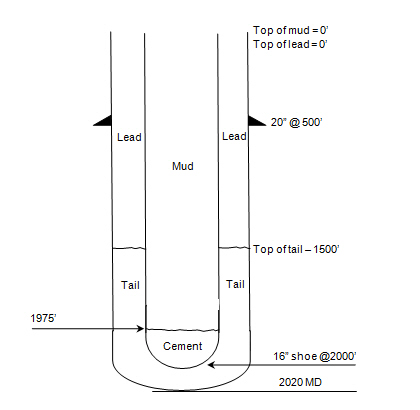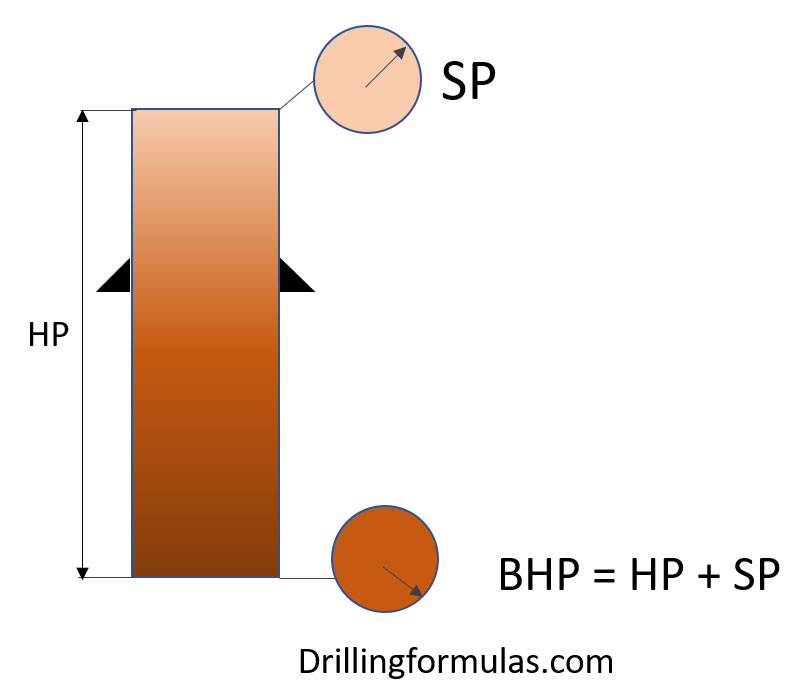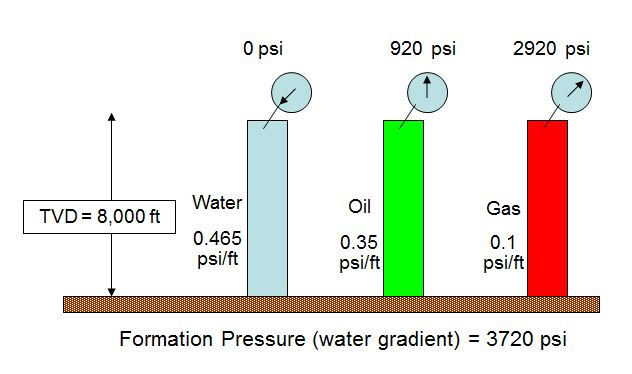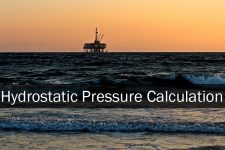## How much pressure will we see at cement head in case of float shoe fail?

I still have the simple but interesting question about hydrostatic pressure which you can apply this calculation into drilling/cementing operation. The question I got about how much pressure we will see at cement head in case of float shoe fail.## Bottom Hole Pressure Relationship

This article will show you about bottom hole pressure relationship because this concept is very important for well control concept. The bottom hole pressure is a summation of all the pressure acting on the bottom hole.

Bottom Hole Pressure (BHP) = Surface Pressure (SP) + Hydrostatic Pressure (HP)

The image below demonstrates the relationship of bottom hole pressure.Note: BHP created by hydrostatic column of drilling fluid is the primary well control in drilling.

Looking more into details,

If BHP is more than FP (formation pressure), this situation is called “Overbalance”.

If BHP is equal to FP (formation pressure), this situation is called “Balance”.

If BHP is less than FP (formation pressure), this situation is called “Underbalance”.

For more understanding, please follow this example below it demonstrates the relationship of BHP, SP and HP.

Bottom Hole Pressure (BHP) = Surface Pressure (SP) + Hydrostatic Pressure (HP)We assume that formation pressure is normal pressure gradient of water (0.465 psi/ft) so formation pressure at 8000’ TVD = 8000 ft x 0.465 psi/ft = 3720 psi. Click here to learn how to calculate hydrostatic pressure in oilfield.

The first case: Hydrostatic column is water which is equal to formation pressure gradient so SP is equal to 0 psi

The second case: BHP is still be water gradient but fluid column is oil (0.35 psi/ft) which is lower density than water gradient (0.465 psi/ft). Therefore, in order to balance BHP, we need Surface Pressure (SP) of 920 psi (SP = 3720 – (0.35 x 8000)).

The third case: BHP is still be water gradient but fluid column is gas (0.1 psi/ft) which is even lower density than water gradient (0.465 psi/ft). Therefore, in order to balance BHP, we need Surface Pressure (SP) of 2,920 psi (SP = 3720 – (0.1 x 8000)).

According to the example, Surface Pressure (SP) will compensate the lack of hydrostatic pressure (HP) in order to balance formation pressure (FP).

References

Cormack, D. (2007). An introduction to well control calculations for drilling operations. 1st ed. Texas: Springer.

Crumpton, H. (2010). Well Control for Completions and Interventions. 1st ed. Texas: Gulf Publishing.

Grace, R. (2003). Blowout and well control handbook [recurso electrónico]. 1st ed. Paises Bajos: Gulf Professional Pub.

Grace, R. and Cudd, B. (1994). Advanced blowout & well control. 1st ed. Houston: Gulf Publishing Company.

Watson, D., Brittenham, T. and Moore, P. (2003). Advanced well control. 1st ed. Richardson, Tex.: Society of Petroleum Engineers.

## Hydrostatic Pressure CalculationHydrostatic pressure is pressure created by fluid column and two factors affecting hydrostatic pressure are mud weight and True Vertical Depth.

You can read full details about hydrostatic pressure from this article, Understand Hydrostatic Pressure.

In this article, it will demonstrate how to calculate hydrostatic pressure in different oilfield and metric unit.

### 1. Calculate hydrostatic pressure in psi by using mud weight  in pound per gallon (ppg) and feet as the units of True Vertical Depth.

Hydrostatic pressure equation:

Hydrostatic Pressure (HP) = 0.052 × Mud Weight × True Vertical Depth (TVD)

Where;

Mud Weight in ppg

True Vertical Depth in ft

Example;

Mud Weight = 12.0 ppg
True Vertical Depth (TVD) = 10,000 ft
Hydrostatic Pressure (HP) = 0.052 × 12.0 × 10,000
Hydrostatic Pressure (HP) = 6,240 psi

### 2. Calculate hydrostatic pressure in psi by using pressure gradient in psi/ft and feet as the units of True Vertical Depth.

Hydrostatic pressure equation:

Hydrostatic Pressure (HP) = Pressure gradient in psi/ft × True Vertical Depth (TVD)

Where;

Pressure gradient in psi/ft

True Vertical Depth in ft

Example;

Pressure Gradient = 0.5 psi/ft
True Vertical Depth (TVD) = 10,000 ft
Hydrostatic Pressure (HP) = 0.5 psi/ft × 10,000 ft
Hydrostatic Pressure (HP) = 5,000 psi

### 3. Calculate hydrostatic pressure in psi by using mud weight in lb/ft3 and feet as the units of True Vertical Depth.

Hydrostatic pressure equation:

Hydrostatic Pressure (HP) = 0.006944 × Mud Weight × True Vertical Depth (TVD)

Where;

Mud Weight in lb/ft3

True Vertical Depth in ft

Example;

Mud Weight = 80 lb/ft3
True Vertical Depth (TVD) = 10,000 ft
Hydrostatic Pressure (HP) = 0.006944 × 80 lb/ft3 × 10,000 ft
Hydrostatic Pressure (HP) = 5,555 psi

### 4. Calculate hydrostatic pressure in psi by using mud weight in PPG and meters as unit of True Vertical Depth.

Hydrostatic pressure equation:

Hydrostatic Pressure (HP) = Mud Weight  × 0.052 × TVD in meters x 3.281

Where;

Mud Weight in ppg

True Vertical Depth in meter

Example:

Mud Weight = 12.0 ppg
True Vertical Depth (TVD) = 5000 meters
Hydrostatic Pressure (HP) = 12.0 × 0.052 × 5000 × 3.281
Hydrostatic Pressure (HP) = 10,237 psi

### 5. Calculate hydrostatic pressure in Pascal by using mud weight  in kg per cu-meter (kg/m3) and meter (m) as the units of True Vertical Depth.

Hydrostatic Pressure (HP) = 9.81 × Mud Weight × True Vertical Depth (TVD)

Where;

Mud Weight in kg/m3

True Vertical Depth in meter

Example;

Mud Weight = 1,000 kg/m3
True Vertical Depth (TVD) = 100 m
Hydrostatic Pressure (HP) = 9.81 × 1,000 × 100
Hydrostatic Pressure (HP) = 981,000 pascal

### 6. Calculate hydrostatic pressure in bar by using mud weight  in kg per cu-meter (kg/m3) and meter (m) as the units of True Vertical Depth.

Hydrostatic Pressure (HP) = 9.81 × Mud Weight × True Vertical Depth (TVD) ÷ 100,000

Where;

Mud Weight in kg/m3

True Vertical Depth in meter

Example;

Mud Weight = 1,000 kg/m3
True Vertical Depth (TVD) = 100 m
Hydrostatic Pressure (HP) = 9.81 × 1,000 × 100 ÷ 100,000
Hydrostatic Pressure (HP) = 9.81 bar

### 7ุ. Calculate hydrostatic pressure in atm by using mud weight  in kg per cu-meter (kg/m3) and meter (m) as the units of True Vertical Depth.

Hydrostatic Pressure (HP) = 9.81 × Mud Weight × True Vertical Depth (TVD)  × 9.8692 × 10-6

Where;

Mud Weight in kg/m3

True Vertical Depth in meter

Example;

Mud Weight = 1,000 kg/m3
True Vertical Depth (TVD) = 100 m
Hydrostatic Pressure (HP) = 9.81 × 1,000 × 100 × 9.8692 × 10-6
Hydrostatic Pressure (HP) = 9.6817 atm